[BACK]
 Intelligent Automation & Soft ComputingDOI:10.32604/iasc.2022.023322Article

Exact Run Length Evaluation on Extended EWMA Control Chart for Autoregressive Process

Department of Applied Statistics, Faculty of Applied Science, King Mongkut’s University of Technology NorthBangkok, Bangkok, 10800, Thailand
*Corresponding Author: Yupaporn Areepong. Email: yupaporn.a@sci.kmutnb.ac.th
Received: 03 September 2021; Accepted: 16 November 2021

Abstract: Extended Exponentially Weighted Moving Average (Extended EWMA or EEWMA) control chart is one of the control charts which can quickly detect a small shift. The average run length (ARL) measures the performance of control chart. Due to the derivation of the explicit formulas for ARL on the EEWMA control chart for the autoregressive AR(p) process has not previously been reported. The aim of the article is to derive explicit formulas of ARL using a Fredholm integral equation of the second kind on EEWMA control chart for Autoregressive process, as AR(2) and AR(3) processes with exponential white noise. The accuracy of the solution obtained with the EEWMA control chart was compared to the numerical integral equation (NIE) method and extended to compare performance with Cumulative Sum (CUSUM) and Exponentially Weighted Moving Average (EWMA) control charts. The results show that the ARL obtained by the explicit formula and the NIE method are hardly different but ARL of explicit formula is less the computational (CPU) time than ARL of NIE method. The performance of EEWMA control chart is better than the CUSUM and EWMA control charts for all situations except when the large shift sizes the EEWMA control chart performed as well as the EWMA control chart for AR(2) and AR(3) processes. And then, the EEWMA control chart is also extended to compare efficiency of EEWMA control chart with various λ. An exponential smoothing parameter of 0.05 is recommended. In addition, the simulation study, and efficacy illustration with real data on new COVID-19 cases in Thailand and Vietnam provided similar results.

Keywords: Extended EWMA control chart; autoregressive process; average run length; explicit formula

1  Introduction

Currently, the statistical process control (SPC) is very important in the manufacturing industry for monitoring, controlling, and improving processes. Control charts are one of the efficient tools of SPC and have been applied in many fields such as finance , health , and medicine . The Shewhart control chart was the first to be reported and is widely used for detecting large changes in a process mean . Subsequently, the Cumulative Sum (CUSUM) chart  and the Exponentially Weighted Moving Average (EWMA) chart  have been widely employed to monitor a process mean due to their excellent performance in detecting small to moderate mean shifts. In addition, Patel et al. -proposed the Modified Exponentially Weighted Moving Average (Modified EWMA) chart that is effective at detecting small size shift quickly for observations both autocorrelation and independently normally distribution. Later, Neveed et al.  proposed the Extended Exponentially Weighted Moving Average (EEWMA) chart that performed better than other control charts for detecting small shifts in the mean of a monitored process.

The performance of the chart is measured by Average Run Length (ARL). The ARL0 denote the average number of observations before an in-control process is taken to signal to be out of control and should be large whereas the ARL1 denote the average number of observations taken from out of control and should be as small as possible.

Many methods for evaluating ARL for control charts have been studied. For example, Monte Carlo simulations (MC), Markov Chain approach (MCA), Martingale approach (MA) and Numerical Integral Equation approach (NIE) and explicit formulas. Mastrangelo et al.  evaluated ARL of the traditional EWMA chart for serially correlated processes by using the Monte Carlo simulation method. Zhang et al.  proposed the ARL of the multivariate exponentially weighted moving average (MEWMA) chart and the combined control chart were evaluated with Monte Carlo simulation. Sukparungsee  approximated the ARL with optimal parameters of one and two-sided EWMA control chart using by Martingale approach. Chananet et al.  evaluated the ARL of EWMA and CUSUM control charts with Markov Chain approach based on the zero-inflated negative binomial (ZINB) model.

Many literatures for evaluating the ARL using NIE method and explicit formula have been studied. Areepong et al.  proposed the ARL using the numerical integral equation approach of the EWMA chart and compared the results with the Monte Carlo simulation method. Khoo et al.  presented a Markov chain approach for computing the ARL of EWMA charts. Moreover, Phanyaem et al.  derived the ARL for ARMA processes via explicit formula and numerical integral equation (NIE) method of EWMA chart. Petcharat et al.  investigated the derivation of the ARL for moving average order q process with exponential white noise by explicit formula. After that, Peerajit et al.  studied the NIE method of ARL on CUSUM chart. Supharakonsakun et al.  evaluated the ARL by NIE method on modified EWMA and compared efficiency with EWMA control chart. Sunthornwat et al.  derived explicit formulas of ARL on CUSUM chart for seasonal and non-seasonal moving average processes with exogenous variables and evaluated against the NIE method. Later, Anwar et al.  proposed modified-mxEWMA chart that performs very well for the monitoring of small to moderate shifts in the process and show the implementation of the wood industry. Saghir et al.  proposed modified EWMA chart and the performance is evaluated by ARL. Aslam et al.  proposed new Bayesian Modified-EWMA chart and its applications in mechanical and sport industry. Karoon et al.  developed the numerical integral equation (NIE) methods for evaluating the ARL on Extended EWMA chart for AR(p) process. Supharakonsakun et al.  presented the exact average run length based on explicit formula the observations are from moving average process with exponential white noise for modified EWMA chart. Phanthuna et al.  proposed the explicit formula for evaluating the ARL on a two-sided modified EWMA chart under the observations of AR(1) process. Recently, Phanthuna et al.  presented explicit formula of ARL for modified EWMA chart with autoregressive model involving exponential white noise.

However, the derivation of the explicit formulas for ARL on the EEWMA chart for autoregressive AR(p) process has not previously been reported. Therefore, the aim of this study is to derive explicit formulas of the ARL on the EEWMA control chart for AR(p) process, as AR(2) and AR(3) processes with exponential white noise. The explicit formulas for ARL were compared with the (NIE) method as the benchmark. Besides, the performance of the explicit formulas for deriving the ARL on the EEWMA chart was compared with those on the CUSUM and EWMA charts for both simulated data and real-world data reported.

2  Materials and Methods

2.1 Cumulative Sum (CUSUM) Control Chart

The CUSUM control chart was originally introduced by Page  in quality control to detect small changes in process mean, as an extension of Shewhart control chart. The CUSUM control chart can be expressed by the recursive equation below.

Ct=max(0,Ct1+Xta),t=1,2, (1)

where a is non-zero constant, C0 is the initial value of CUSUM statistics with u ∈ [0, b], C0 = u.

The stopping time of the CUSUM control chart is given by

τb=inf{t > 0;Ct > b},b > u (2)

where τb is the stopping time, b is upper control limit (UCL).

2.2 Exponentially Weighted Moving Average (EWMA) Control Chart

The EWMA control chart was initially proposed by Robert . It is usually used to monitor and detect small changes in process mean. The EWMA control chart can be expressed by the recursive equation below.

Zt=(1λ)Zt1+λXt,t=1,2, (3)

where Xt is a process with mean, λ is an exponential smoothing parameter with 0 < λ < 1 and Z0 is the initial value of EWMA statistics, Z0 = u. The upper control limit (UCL) and Lower control limit (LCL) of EWMA control charts are given by

UCL=μ0+Qσλ2λ, (4)

LCL=μ0Qσλ2λ, (5)

where μ0 is the target mean, σ is the process standard deviation, and Q is suitable control limit width.

The stopping time of the EWMA control chart is given by

τh=inf{t0:Zt > h},h > u (6)

where τh is the stopping time, h is UCL.

2.3 Extended Exponentially Weighted Moving Average (Extended EWMA or EEWMA) Control Chart

The EEWMA control chart was proposed by Neveed et al. . It is developed from the EWMA control chart. This is effective to monitored and detected small changes in process mean. The EWMA control chart can be expressed by the recursive equation below.

Et=λ1Xtλ2Xt1+(1λ1+λ2)Et1,t=1,2,, (7)

where λ1 and λ2 are exponential smoothing parameters with (0 < λ1 ≤ 1) and (0 ≤ λ2 < λ1) and the initial value is a constant, E0 = u. The upper control limit (UCL) and Lower control limit (LCL) of the EEWMA control charts are given by

UCL=μ0+Lσλ12+λ222λ1λ2(1λ1+λ2)2(λ1λ2)(λ1λ2)2, (8)

LCL=μ0Lσλ12+λ222λ1λ2(1λ1+λ2)2(λ1λ2)(λ1λ2)2, (9)

where μ0 is the target mean, σ is the process standard deviation, and L is suitable control limit width.

The stopping time of the EEWMA control chart is given by

τh=inf{t0:Et > h},h > u (10)

where τh is the stopping time, h is UCL.

3  Explicit Formulas of ARL on the EEWMA Control Chart for AR(p) Processes

Let L(u) denote the ARL for the autoregressive process, to define function L(u) as

ARL=L(u)=Eθ(τb)T (11)

where Eθ() is the expectation under the assumption that the change point occurs at time θ and θ is the change point time.

The equation of observations for autoregressive (AR(p)) process in the case of an exponential while noise denoted can be described by

Xt=η+ϕ1Xt1+ϕ2Xt2++ϕpXtp+ϵt (12)

where Xt (t = 1, 2, 3, …) is a sequence of random variables, η is a suitable constant, Φ is an autoregressive coefficient (− 1 ≤ Φ ≤ 1), and ɛt is white noise sequence of exponential (ɛt ∼ Exp(α)). The probability density function of ɛt is given by f(x)=1αexα where x ≥ 0.

Let L(u) denote ARL for AR(p) process, the EEWMA statistics Et can be written as:

Et=(1λ1+λ2)Zt1+(λ1ϕ1λ2)Xt1+λ1ϕ2Xt2+λ1ϕ3Xt3++λ1ϕpXtp+λ1η+λ1ϵt

where (0 < λ1 ≤ 1), (0 ≤ λ2 < λ1) and the initial value E0 = u, and Xt−1, Xt−2, …, Xtp.

Consequently, the EEWMA statistics Et can be written as

Et=(1λ1+λ2)u+(λ1ϕ1λ2)Xt1+λ1ϕ2Xt2+λ1ϕ3Xt3++λ1ϕpXtp+λ1η+λ1ϵt

If ɛt = 0 LCL = 0 and UCL = h, respectively. Then

0Eth

0(1λ1+λ2)u+(λ1ϕ1λ2)Xt1+λ1ϕ2Xt2+λ1ϕ3Xt3++λ1ϕpXtp+λ1η+λ1ϵth

0(1λ1+λ2)u(λ1ϕ1λ2)Xt1λ1ϕ2Xt2λ1ϕpXtpλ1ηεth'(1λ1+λ2)u(λ1ϕ1λ2)Xt1λ1ϕ2Xt2λ1ϕpXtpλ1η

Let L(u) denote the ARL on the EEWMA control chart. The function L(u) can be derived by Fredholm integral equation of the second kind, L(u) is defined as follows:

L(u)=1+L(E1)f(ϵ1)dϵ1 (13)

Therefore, the function L(u) is obtained as follows:

L(u)=1+0(1λ1+λ2)u(λ1ϕ1λ2)Xt1λ1ϕ2Xt2λ1ϕpXtpλ1ηh(1λ1+λ2)u(λ1ϕ1λ2)Xt1λ1ϕ2Xt2λ1ϕpXtpλ1ηL((1λ1+λ2)u+(λ1ϕ1λ2)Xt1+λ1ϕ2Xt2++λ1ϕpXtp+λ1η+λ1y)f(y)dy

If k = (1 − λ1 + λ2)u + (λ1Φ1 − λ2)Xt−1 + λ1Φ2Xt−2 + … + λ1ΦpXtp + λ1η + λ1y is defined for changing the integration variable, the function L(u) is given by

L(u)=1+1λ10hL(k)f(k(1λ1+λ2)u(λ1ϕ1λ2)Xt1λ1ϕ2Xt2ϕpXtpη)dk (14)

The L(u) is Fredholm integral equation of the second kind. If ɛt ∼ Exp(α), then

L(u)=1+1λ1α0hL(k)ekλ1αe(1λ1+λ2)u+(λ1ϕ1λ2)Xt1λ1α+ϕ2Xt2++ϕpXtp+ηαdk

L(u)=1+e(1λ1+λ2)u+(λ1ϕ1λ2)Xt1λ1α+ϕ2Xt2++ϕpXtp+ηαλ1α0hL(k)ekλ1αdk (15)

WhenG(u)=e(1λ1+λ2)u+(λ1ϕ1λ2)Xt1λ1α+ϕ2Xt2++ϕpXtp+ηα,F=0hL(k)ekλ1αdk,

Consequently,L(u)=1+G(u)λ1αF. (16)

Consider the constant F and take turn L(k) with Eq. (16), then

F=0hL(k)ekλ1αdk

=1+0h[1+G(k)λ1αF]ekλ1αdk

=0hekλ1αdk+0hG(k)λ1αFekλ1αdk

=λ1α(ehλ1α1)Fλ1λ2e(λ1ϕ1λ2)Xt1λ1α+ϕ2Xt2++ϕpXtp+ηα(e(λ1λ2)hλ1α1)

F=λ1α(ehλ1α1)1+1λ1λ2e(λ1ϕ1λ2)Xt1λ1α+ϕ2Xt2++ϕpXtp+ηα(e(λ1λ2)hλ1α1) (17)

Finally, substituting constant F form Eq. (17) into Eq. (16), then L(u) can be written as

L(u)=1(λ1λ2)e(1λ1+λ2)uλ1α(ehλ1α1)(λ1λ2)e{(λ1ϕ1λ2)Xt1λ1α+ϕ2Xt2++ϕpXtp+ηα}+(e(λ1λ2)hλ1α1). (18)

The process is “in-control” with the exponential parameter α = α0, the explicit formula of the ARL0 for AR(p) process on the EEWMA control chart can be written as follows:

ARL0=1(λ1λ2)e(1λ1+λ2)uλ1α0(ehλ1α01)(λ1λ2)e{(λ1ϕ1λ2)Xt1λ1α0+ϕ2Xt2++ϕpXtp+ηα0}+(e(λ1λ2)hλ1α01). (19)

Meanwhile, the process is “out-of-control” with the exponential parameter α = α1 and then α1 = (1 + δ)α0, where α1 > α0 and δ is the shift size, the explicit formula of ARL1 for AR(p) process on the EEWMA control chart can be written as follows:

ARL1=1(λ1λ2)e(1λ1+λ2)uλ1α1(ehλ1α11)(λ1λ2)e{(λ1ϕ1λ2)Xt1λ1α1+ϕ2Xt2++ϕpXtp+ηα1}+(e(λ1λ2)hλ1α11). (20)

while (− 1 ≤ Φ ≤ 1) is the autoregressive coefficient, (0 < λ1 ≤ 1), (0 ≤ λ2 < λ1) are the smoothing parameters, the initial value E0 = u, and Xt−1, Xt−2, …, Xtp and h is the upper control limit.

4  Numerical Integral Equation Method of ARL on the EEWMA Control Chart for AR(p) Processes

The NIE method is used to solve the ARL for the AR(p) process on the EEWMA control chart in Eq. (14). The ARL solution or L~(u) is approximated with the m linear equation systems over the interval [0, h]. A quadrature rule is used to approximate the integral by a finite sum of areas of rectangles with base h/m and heights chosen as the values of f(aj) at the midpoints of intervals of length beginning at zero with a set of constant weights wj=hm;j=1,2,,m and aj=hm(j12) (see ).

Therefore, the approximating NIE method for the ARL on the EEWMA control chart is evaluated as follows:

0hL(k)f(k)dkj=1mwjf(aj) (21)

The system of m linear equation is showed as:

Lm×1 = 1m×1 + Rm×mLm×1 or (Im − Rm×m)Lm×1 = 1m×1 or Lm×1 = (Im − Rm×m)−11m×1

Lm×1 = (Im − Rm×m)−11m×1 where Lm×1=[L~(a1),L~(a2),,L~(am)]T , Im = diag(1, 1, …, 1) and 1m×1 = [1, 1, …, 1] T.

Let Rm×m be a matrix, the definition of the m to mth element of the matrix R is given by

[Rij]1λ1wjf(aj(1λ1+λ2)ai(λ1ϕ1λ2)Xt1λ1ϕ2Xt2ϕpXtpη)

Finally, the numerical approximation for the function L~(u) is as follows:

L~(u)=1+1λ1j=1mwjL(aj)f(aj(1λ1+λ2)u(λ1ϕ1λ2)Xt1λ1ϕ2Xt2ϕpXtpη) (22)

5  Existence and Uniqueness of ARL

The solution of ARL shows that there uniquely exists the integral equation for explicit formulas by the Banach’s Fixed-point Theorem. In this study, let T be an operation in the class of all continuous functions defined by

T(L(u))=1+1λ10hL(k)f(k(1λ1+λ2)u(λ1ϕ1λ2)Xt1λ1ϕ2Xt2ϕpXtpη)dk (23)

According to Banach’s Fixed-point Theorem, if an operator T is a contraction, and then the fixed-point equation T(L(u)) = L(u) has a unique solution. To show that Eq. (23) exists and has a unique solution, theorem can be used as follows below.

Theorem 1 Banach’s Fixed-point Theorem: Let (X, d) be a complete metric space and T: X → X be a contraction mapping with contraction constant 0 ≤ r < 1 such that ‖T(L1) − T(L2)‖ ≤ rL1 − L2‖, L1,L2X . Then there exists a unique L( ⋅ ) ∈ X such that T(L(u)) = L(u), i.e., a unique fixed-point in X.

Proof: Let T defined in Eq. (23) is a contraction mapping for L1, L2 ∈ G[0, h], such that ‖T(L1) − T(L2)‖ ≤ rL1 − L2‖, L1,L2G[0,h] with 0 ≤ r < 1 under the norm L=supu[0,h]|L(u)| , so

T(L1)T(L2)=supu[0,h]|1λ1αe(1λ1+λ2)u+(λ1ϕ1λ2)Xt1λ1α+ϕ2Xt2++ϕpXtp+ηα0h(L1(k)L2(k))ehλ1αdk|

supu[0,h]|L1L21λ1αe(1λ1+λ2)u+(λ1ϕ1λ2)Xt1λ1α+ϕ2Xt2++ϕpXtp+ηα(λ1α)(ehλ1α1)|=L1L2supu[0,h]|e(1λ1+λ2)u+(λ1ϕ1λ2)Xt1λ1α+ϕ2Xt2++ϕpXtp+ηα||1ehλ1α|

rL1L2

wherer=supu[0,h]|e(1λ1+λ2)u+(λ1ϕ1λ2)Xt1λ1α+ϕ2Xt2++ϕpXtp+ηα||1ehλ1α|;0r<1.

6  Numerical Results

The absolute percentage relative error (APRE) to measure the accuracy of the ARL is defined as

APRE(%)=| L(u)L˜(u) |L(u)×100 (24)

where L(u) is the explicit formulas of the ARL on the EEWMA control chart for AR(p) process shows that Eq. (18) , which ARL0 and ARL1 are Eqs. (19) and (20), respectively, and L~(u) in Eq. (22) is the NIE method of the ARL using the Gauss-Legendre quadrature rule on the EEWMA control chart for AR(p) with the number of division points m =500 nodes. The numerical results were computed by MATHEMATICA. The initial parameter values are studied at ARL0 = 370 on the EEWMA control chart for AR(p) process, referred to as AR(2) and AR(3) processes with exponential white noise and given λ1 = 0.05, 0.10, λ2 = 0.01, 0.02, 0.03. The ‘in-control’ process had parameter value as α = α0 with shift size (δ = 0). On the other hand, the ‘out-of-control’ process was presented with parameter values as α1 = (1 + δ)α0 with shift sizes (δ) equals 0.001, 0.003, 0.005, 0.010, 0.030, 0.050, 0.100, 0.500 and 1.000 were determined. Furthermore, the coefficient parameters of the process Φ1 = 0.2, Φ2 = 0.2, − 0.2 were used for the AR(2) process, and Φ1 = Φ2 = 0.2, Φ3 = 0.2, − 0.2 were used for the AR(3) process. In addition, the speed test results were computed by the CPU time (PC System: windows10, 64-bit, Intel® Core™ i5-8250U 1.60 GHz 1.80 GHz, RAM 4 GB) in seconds.

In Tabs. 1 and 2, the ARL results by using the explicit formula (Eqs. (19) and (20)) and the NIE method (Eq. (22) then the absolute percentage relative error named APRE(%) show that Eq. (24). It showed that the ARL values derived from the explicit formulas give results close to those from the NIE method both AR(2) and AR(3) process. AR(2) process, Tab. 1 showed that the analytical results agree with NIE approximations with APRE(%) less than 0.000239% and CPU time of approximately 2.7–3.5 s whereas the CPU time of the explicit formulas is not much. AR(3) process in Tab. 2 showed that the analytical results agree with NIE approximations with APRE(%) less than 0.000216% and CPU time of approximately 2.8–3.5 s whereas the CPU time of the explicit formulas is not much. The entries inside the parentheses are the CPU time in seconds.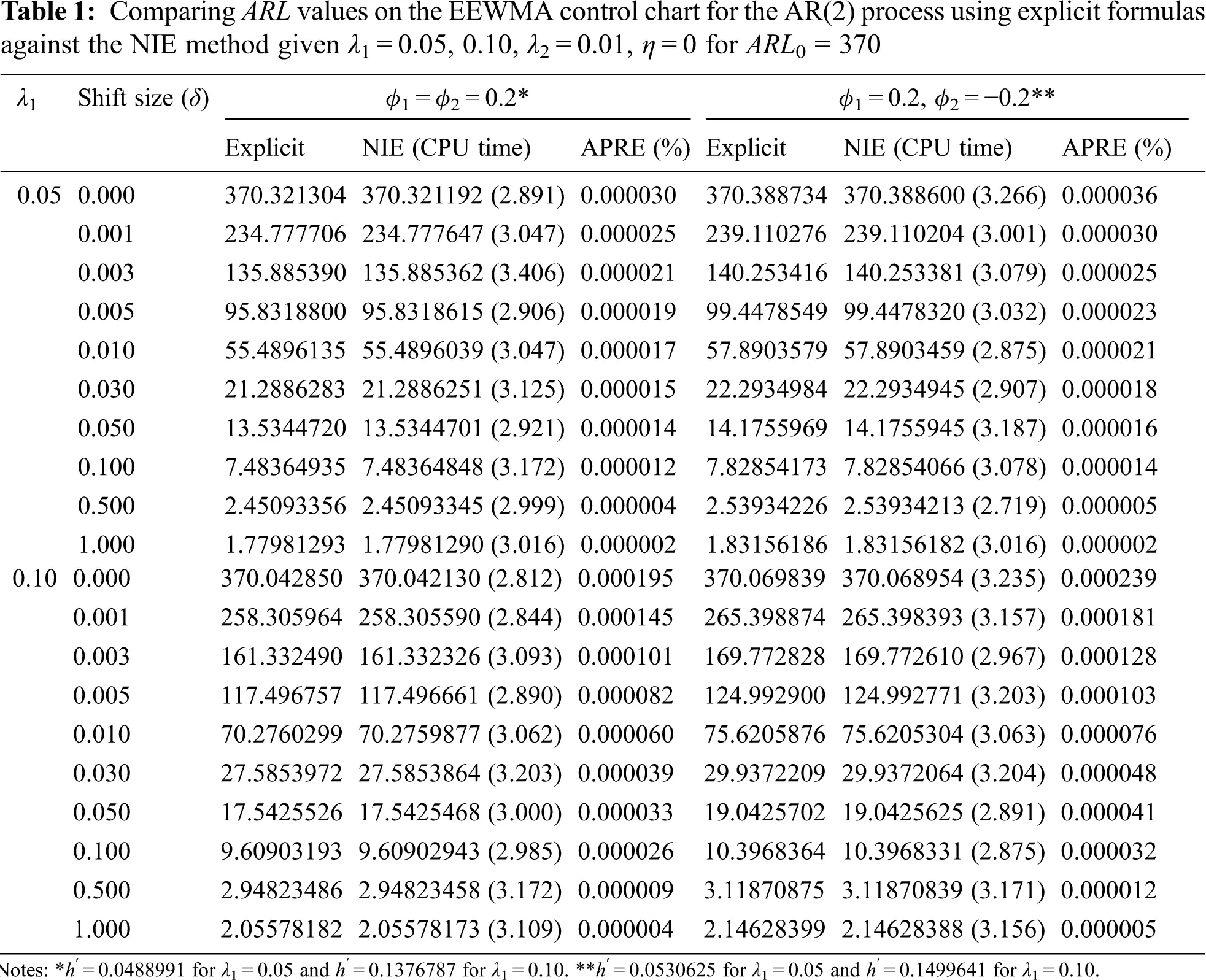7  Performance Comparing the ARL Results

For Tabs. 3 and 4, the EEWMA control chart is compared for various λ1 = 0.05, 0.10 and λ2 = 0.01, 0.02, 0.03 at ARL0 = 370, η = 0, Φ1 = Φ2 = 0.2 (as an AR(2) process) and Φ1 = Φ2 = Φ3 = 0.2 (as an AR(3) process). The ARL values are indicated that the ARL1 on the EEWMA (λ2 = 0.03) or EEWMA_03 control chart was reduced more sensitively than on the EEWMA with either λ2 = 0.01 (EEWMA_01) or λ2 = 0.02 (EEWMA_02) for all magnitudes of changes both AR(2) and AR(3) processes. Moreover, the ARL1 on the EEWMA control chart with λ1 = 0.05 was reduced more sensitively than on the EEWMA control chart with λ1 = 0.10 for all situations running AR(2) and AR(3) processes. The exponential smoothing parameter 0.05 is recommended. Tab. 5 showed that the comparison of ARL values for the AR(2) process on CUSUM, EWMA and EEWMA control charts, the results presented that the ARL1 on the EEWMA control chart with λ2 = 0.03 was reduced the ARL1 more than the CUSUM, EWMA, EEWMA with either λ2 = 0.01 or λ2 = 0.02 control charts for all shift sizes and all exponential smoothing parameter values. Similarly, the ARL results of AR(3) process in Tab. 6, the results presented that the ARL1 on the EEWMA control chart with λ2 = 0.03 was reduced the ARL1 more than the CUSUM, EWMA, EEWMA with either λ2 = 0.01 or λ2 = 0.02 control charts for all shift sizes and all exponential smoothing parameter values as same as the ARL results of AR(2) process. Therefore, the performance of the EEWMA control chart with λ2 = 0.03 is more efficient than the performance of the CUSUM, EWMA, EEWMA with either λ2 = 0.01 or λ2 = 0.02 control charts for all situations except when the large shift sizes (δ ≥ 0.5), the EEWMA control chart with λ2 = 0.03 was reduced as well as the EWMA, EEWMA with either λ2 = 0.01 or λ2 = 0.02 control charts.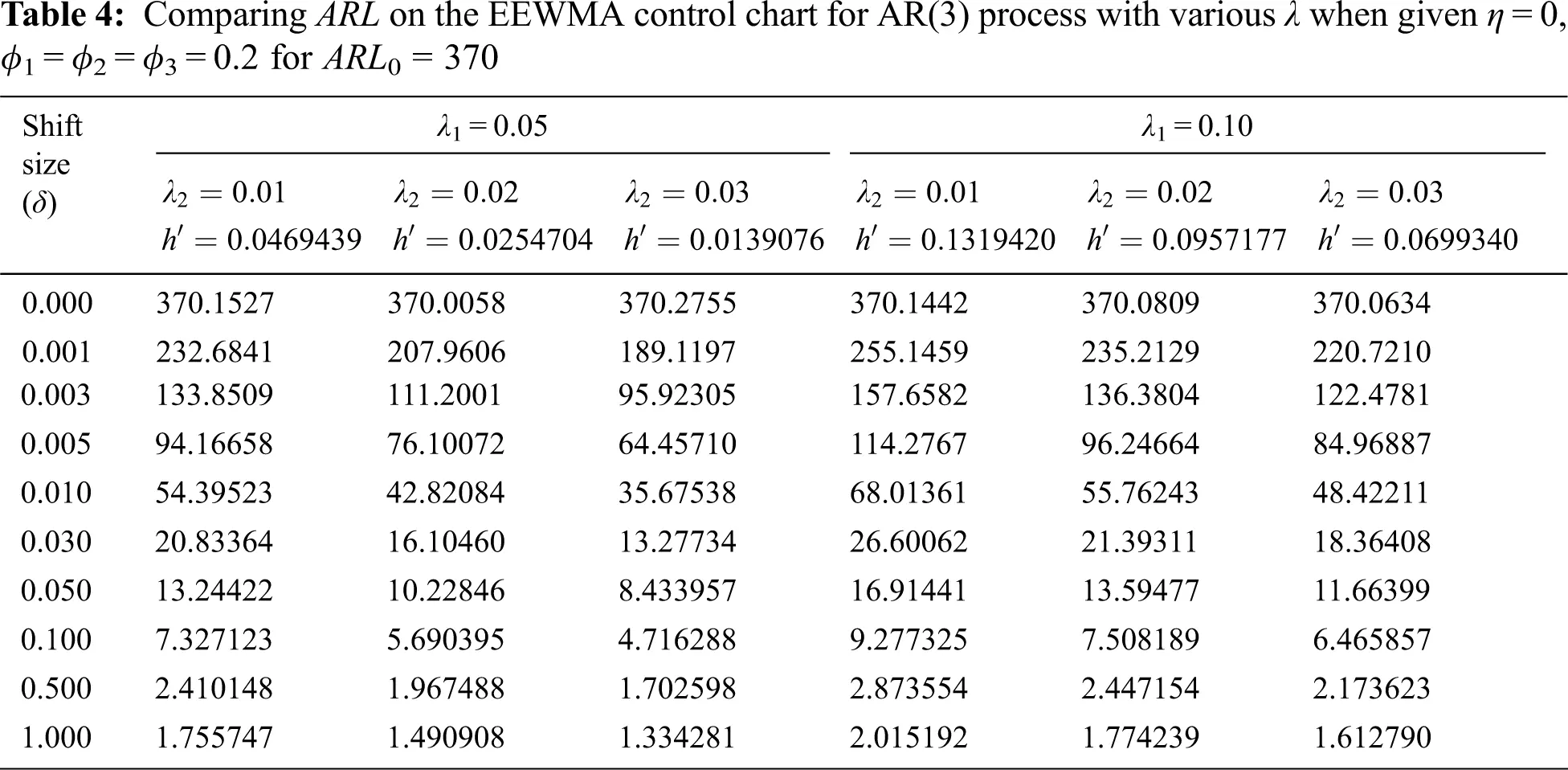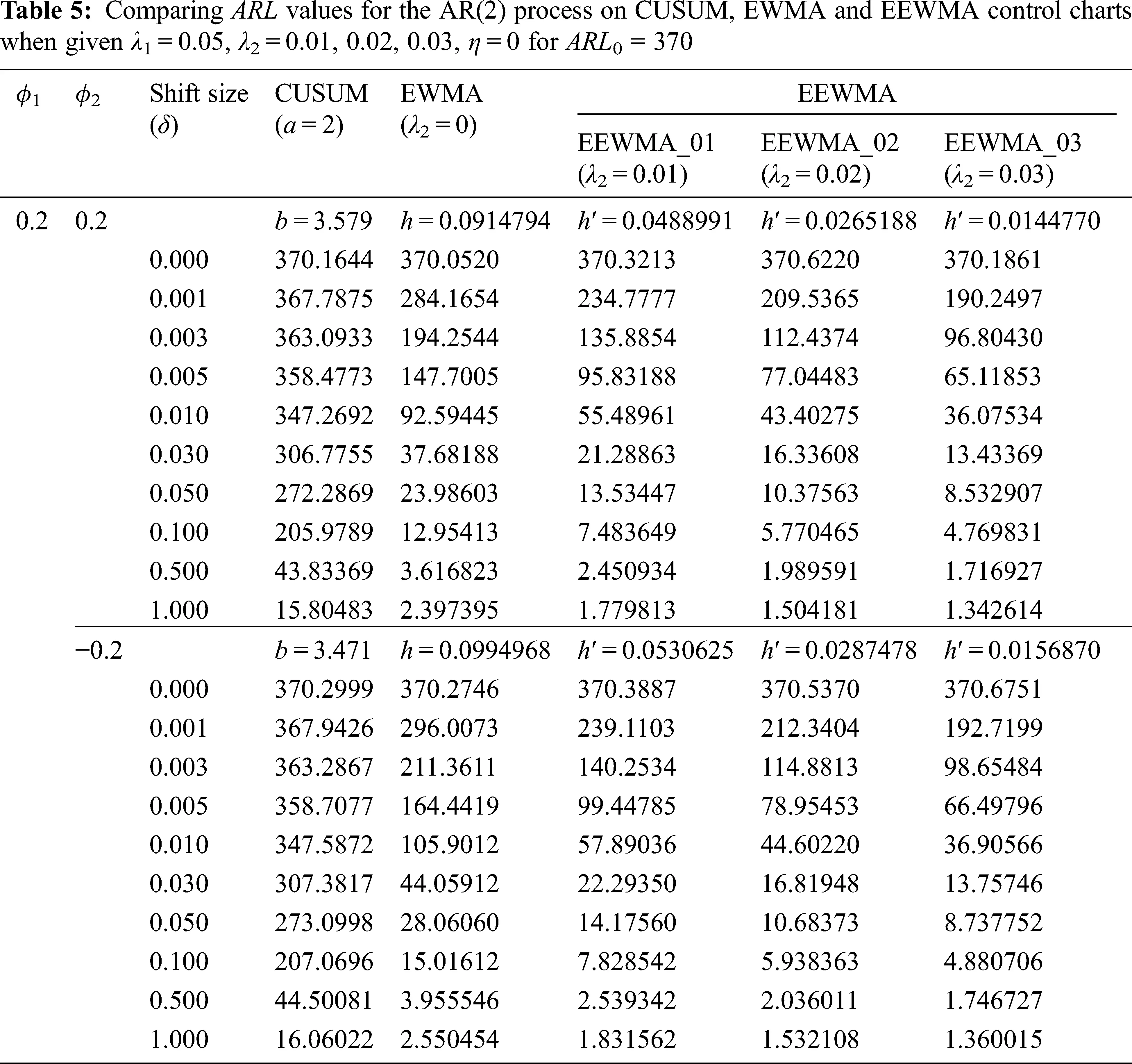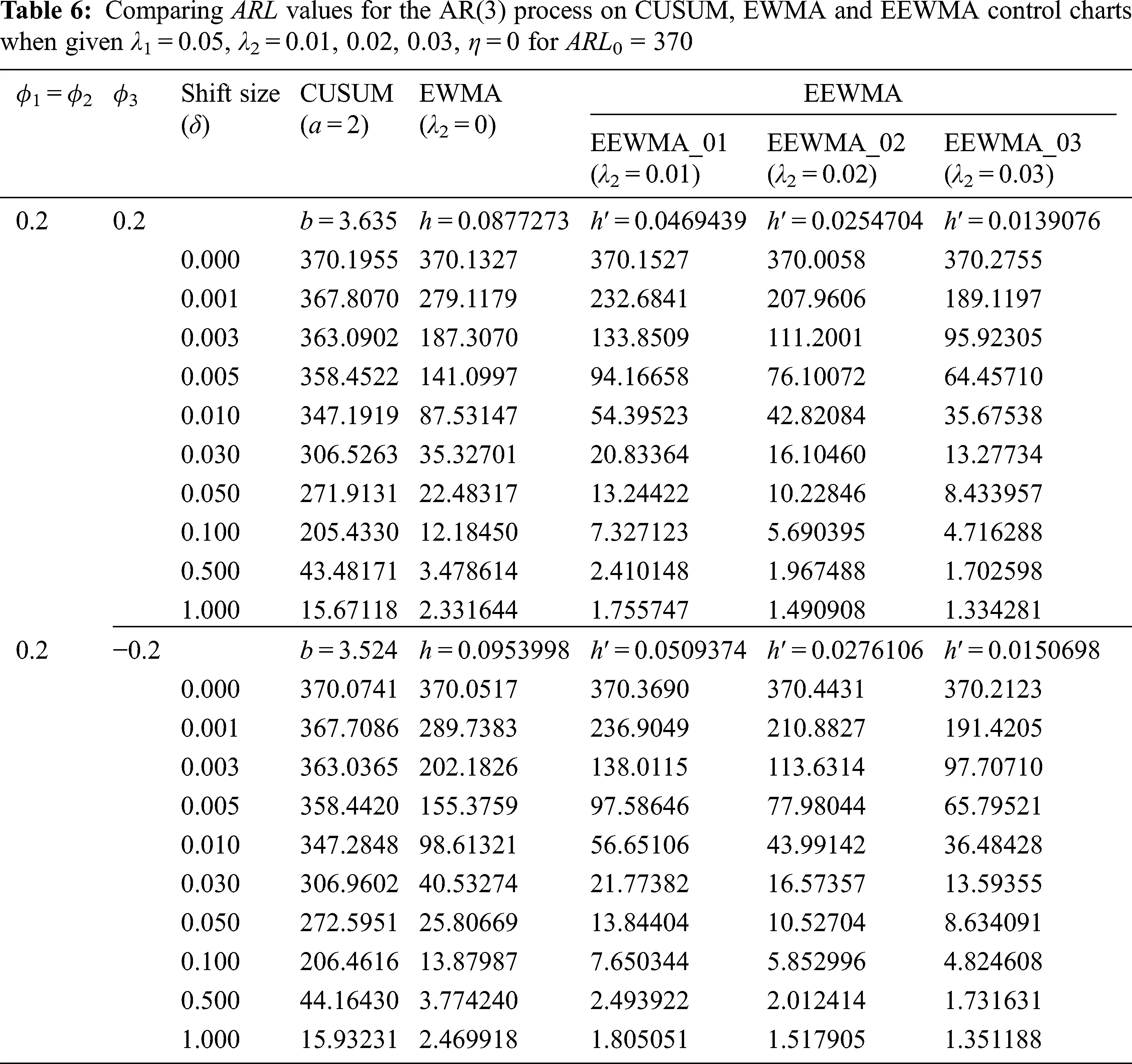8  Application to Real Data

The performance of the ARL constructed using explicit formulas for the EEWMA control chart for λ1 = 0.05 and various λ2 = 0.01, 0.02, 0.03 was compared with those of CUSUM and EWMA (λ2 = 0) control charts using data on new COVID-19 cases in Thailand and in Vietnam from March 30th to July 7th, 2021. Lately, Areepong et al.  investigated monitoring COVID-19 outbreaks in Thailand, Singapore, Vietnam, and Hong Kong using by the EWMA control chart. There are 100 observations of daily. This data is a stationary time series. By looking at the autocorrelation function (ACF) and partial autocorrelation function (PACF). Both countries are the worst affected in Southeast Asia. For λ1 = 0.05 and λ2 = 0.01, 0.02, or 0.03, the settings for the Thailand dataset are that it is an AR(2) process with ARL0= 370; the significance of the mean and standard deviation are 2.774663 and 1.663941, respectively; process coefficients Φ1 = 0.343110, Φ2 = 0.527991; the error is exponential white noise (α0 = 0.665927) whereas the settings for the Vietnam dataset are it is an AR(3) process with ARL0 = 370; the significance of the mean and standard deviation are 0.214103 and 0.259871, respectively; process coefficients Φ1 = 0.269717, Φ2 = 0.572229, Φ3 = 0.219039; the error is exponential white noise (α0 = 0.129397).

The results for the ARL of CUSUM, EWMA and EEWMA with various λ2 = 0.01, 0.02, 0.03 control charts on AR(2) process for the Thailand dataset in Tab. 7 are agreement to the simulation results in Tab. 5. Similarly, These control charts on AR(3) process for the Vietnam dataset in Tab. 8 are agreement to the simulation results in Tab. 6. ARL1 on an EEWMA control chart with λ2 = 0.03 was reduced more sensitively than CUSUM, EWMA, EEWMA with λ2 = 0.01 and EEWMA with λ2 = 0.02 control charts for all magnitudes of changes except when the large shift sizes (δ ≥ 0.5), the EEWMA control chart with λ2 = 0.03 was reduced as well as the EWMA, EEWMA with λ2 = 0.01 and EEWMA with λ2 = 0.02 control charts both AR(2) and AR(3) processes. The results indicate that the performances of the control charts were, in ascending order, EEWMA for λ2 = 0.03, EEWMA for λ2 = 0.02, EEWMA for λ2 = 0.01, EWMA, and CUSUM, as illustrated in Figs. 1 and  2.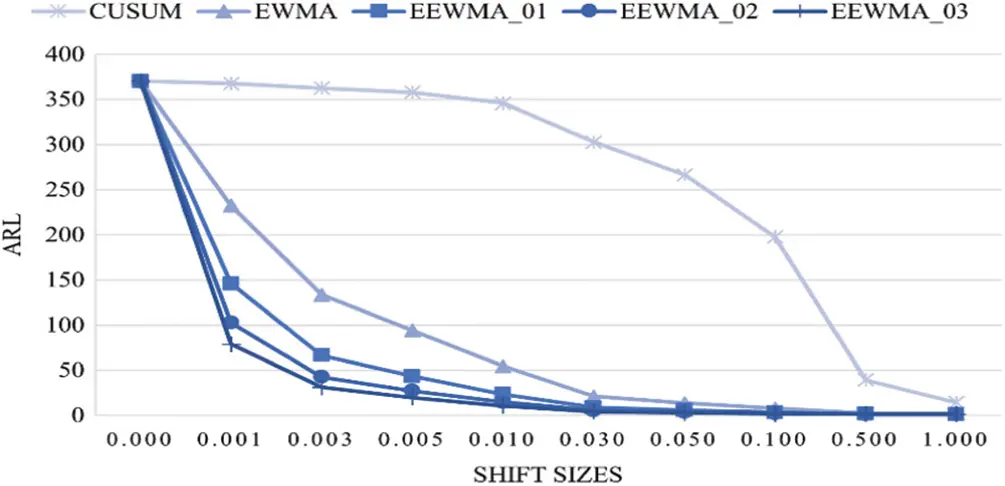Figure 1: ARL values of the AR(2) process on CUSUM, EWMA and EEWMA control charts with new cases COVID-19 data in Thailand when given ARL0 = 370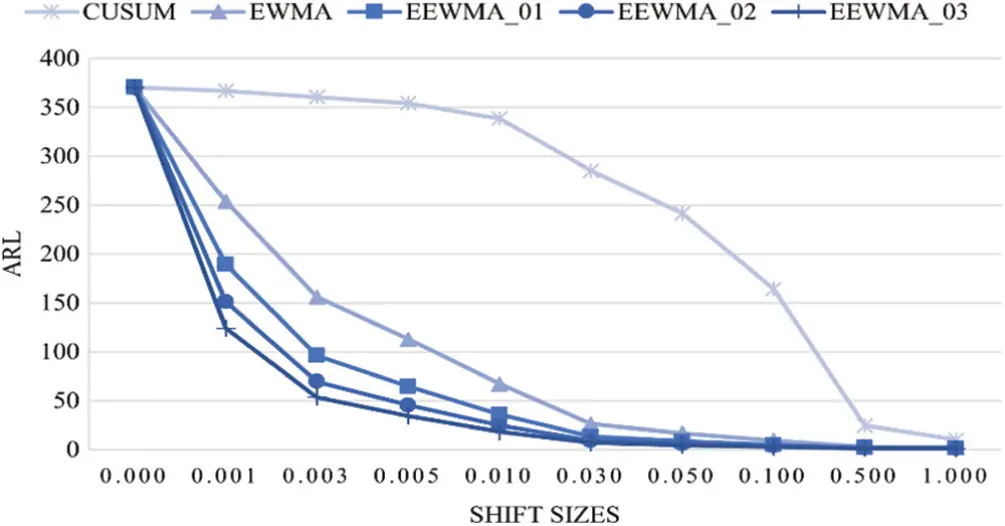Figure 2: ARL values of the AR(3) process on CUSUM, EWMA and EEWMA control charts with new cases COVID-19 data in Vietnam when given ARL0 = 370

As mentioned above, the EEWMA (λ2 = 0.03) and EWMA (λ2 = 0) control charts are plotted by calculating Et and Zt for the two datasets for λ1 = 0.05. The detecting the process with real data of the new cases COVID-19 data in Thailand (as an AR(2) process) and Vietnam (as an AR(3) process) are shown in Figs. 3 and 4, respectively. In Fig. 3, the ARL of the AR(2) process for the Thailand COVID-19 data on the EEWMA control chart for λ2 = 0.03 indicates that the process was signaled as out-of-control at the 6th observation whereas on the EWMA control chart, it was detected at the 11th observation. In Fig. 4 for the EEWMA (λ2 = 0.03) control chart, the ARL of AR(3) process for the Vietnam COVID-19 data on the EEWMA control chart for λ2 = 0.03 was signaled as out-of-control process at the 9th observation whereas on the EWMA control chart, it was detected as out-of-control at the 20th observation. Therefore, the EEWMA control chart can detect shift more quickly than the EWMA control chart.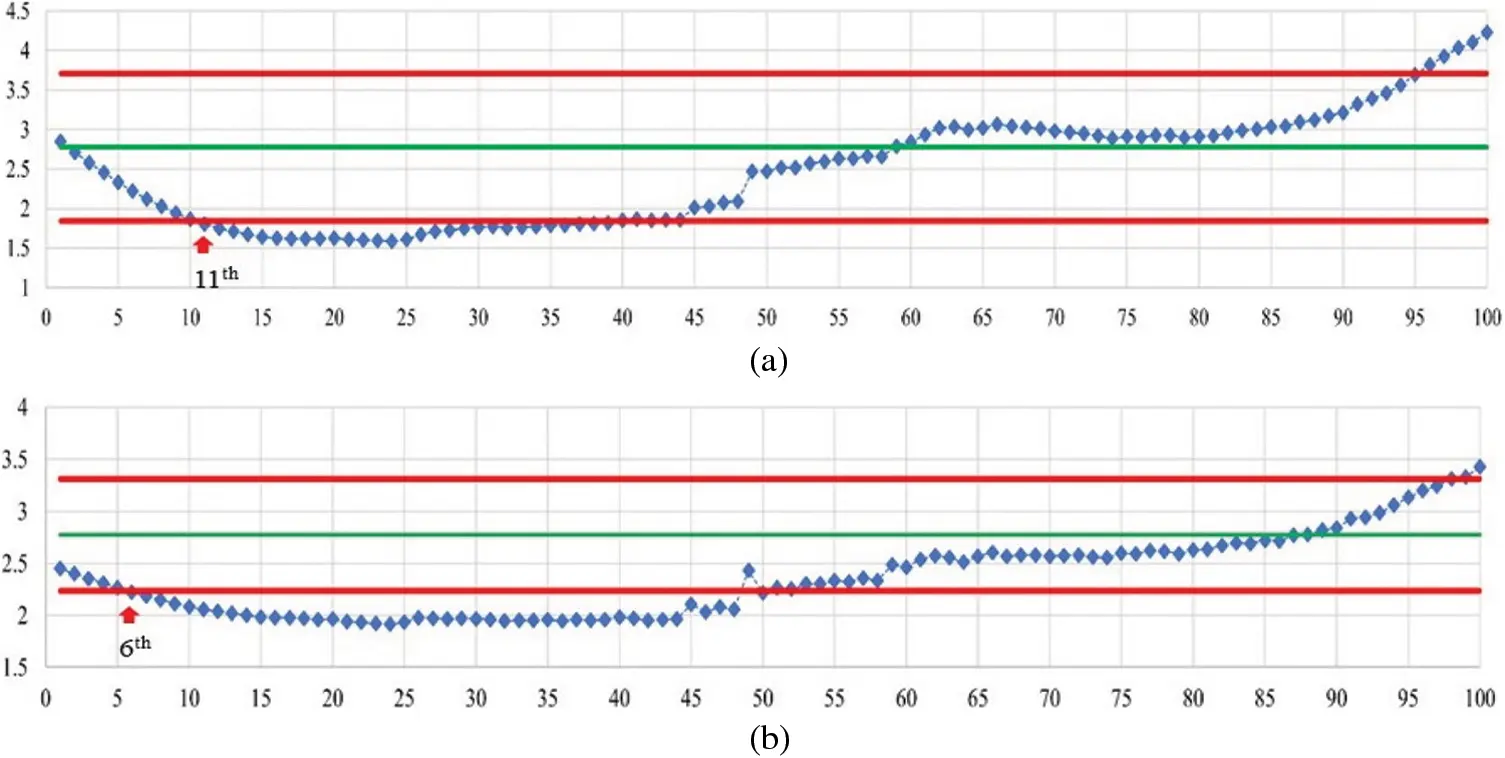Figure 3: The detecting the AR(2) process with the Thailand COVID-19 data when given ARL0 = 370; (a) EWMA control chart and (b) EEWMA control chart at λ2 = 0.03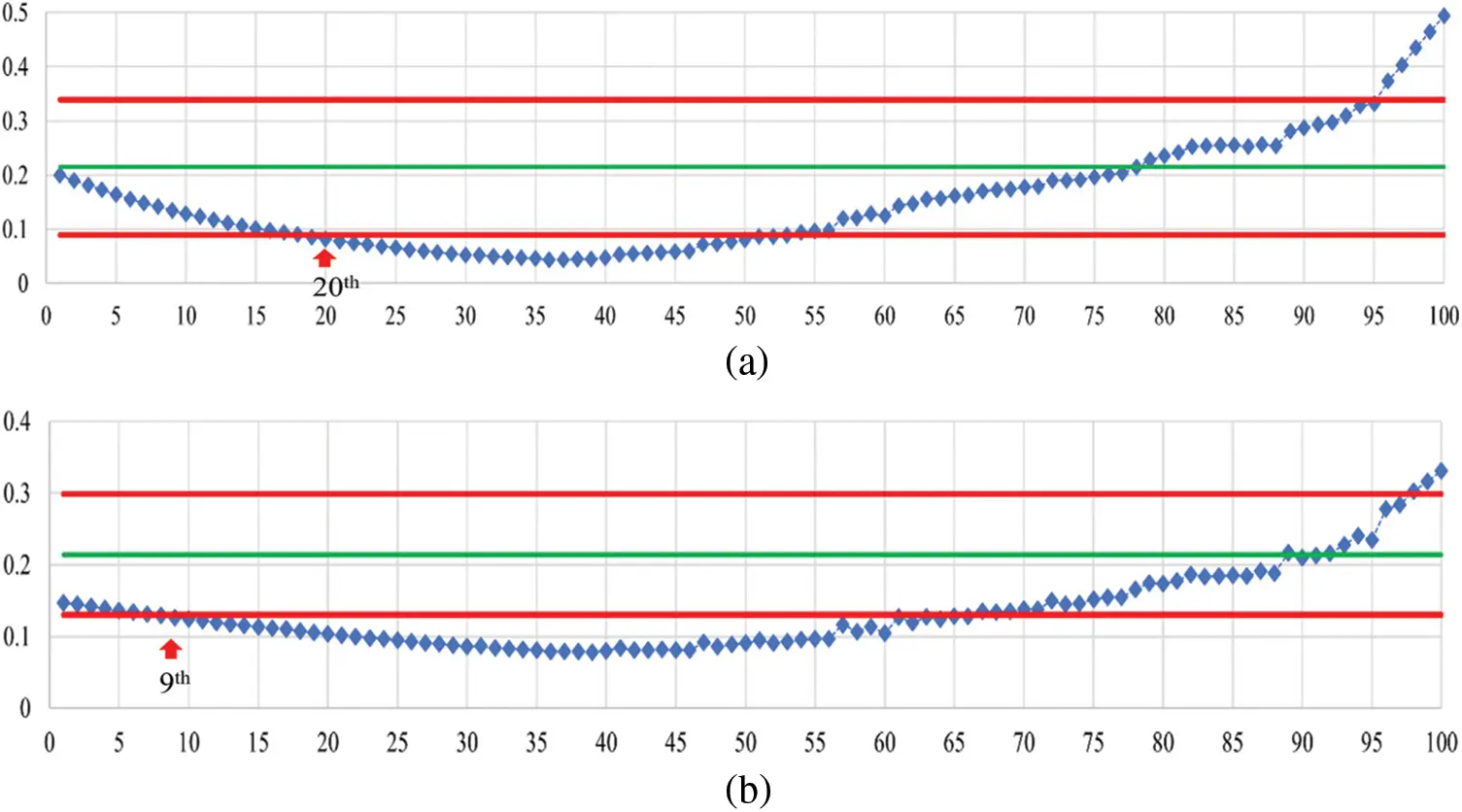Figure 4: The detecting the AR(3) process with the Vietnam COVID-19 data when given ARL0 = 370; (a) EWMA control chart and (b) EEWMA control chart at λ2 = 0.03

9  Discussions and Conclusions

In the study, the performances of control charts were evaluated by using ARL. The explicit formulas comprise a good alternative to the NIE method for constructing the ARL. The analytical results agree with the NIE approximations for AR(2) and AR(3) processes with absolute percentage relative errors of less than 0.000239% and 0.000216%, respectively. The CPU time to calculate the ARL by using the NIE methods were approximately 2.7–3.5 and 2.8–3.5 s for the AR(2) and AR(3) processes, respectively, whereas they were almost instantaneous when using the explicit formulas. The performance comparison of the ARL using explicit formulas on the EEWMA with various λ performed better than on the CUSUM and EWMA control charts running AR(2) or AR(3) processes for most cases except for large shift sizes (δ ≥ 0.5) when even then, the EEWMA control chart with various λ performed as well as the EWMA control chart. The EEWMA control chart with λ2 = 0.03 performed better than the EEWMA with either λ2 = 0.01 or λ2 = 0.02, CUSUM, or EWMA control charts for most magnitudes of changes except for a large shift sizes (δ ≥ 0.5) when it performed at least as well as the others for AR(2) and AR(3) processes. Besides, an exponential smoothing parameter value of 0.05 is recommended. In addition, the simulation study, and the efficacy illustration with real data of new COVID-19 cases in Thailand and Vietnam provided similar results.

Funding Statement: Thailand Science Research and Innovation Fund, and King Mongkut’s University of Technology North Bangkok Contract no. KMUTNB-FF-65–45.

Conflicts of Interest: The authors declare that they have no conflicts of interest to report regarding the present study.

## References

1. M. Kovarik, L. Sarga and P. Klimek, “Usage of control charts for time series analysis in financial management,” Business Economics and Management, vol. 16, no. 1, pp. 138–158, 2015.
2. S. Ozilgen, “Statistical quality control charts: New tools for studying the body mass index of populations form the young to the elderly,” Nutrition, Health & Aging, vol. 15, no. 5, pp. 333–334, 2011.
3. R. Poovarasan, S. Keerthi, K. Yuvashree and C. Thirumalai, “Analysis on diabetes patients using Pearson, cost optimization, control chart” in Proc. of the Int. Conf. on Trends in Electronics and Informatics, Tirunelveli, India, pp. 1139–1142, 2018.
4. W. A. Shewhart, Economic Control of Quality of Manufactured Product. NY, USA: Van Nostrand, 1931.
5. E. S. Page, “Continuous inspection schemes,” Biometrika, vol. 41, no. 1–2, pp. 100–115, 1954.
6. W. S. Roberts, “Control chart tests based on geometric moving averages,” Technometrics, vol. 1, no. 3, pp. 239–250, 1959.
7. A. K. Patel and J. Divecha, “Modified exponentially weighted moving average (EWMA) control chart for an analytical process data,” Chemical Engineering and Materials Science, vol. 2, pp. 12–20, 2011.
8. M. Neveed, M. Azam, N. Khan and M. Aslam, “Design a control chart using extended EWMA statistic,” Technologies, vol. 6, pp. 108–122, 201
9. C. M. Mastrangelo and D. C. Montgomery, “SPC with correlated observations for the chemical and process industried,” Quality and Reliability Engineering International, vol. 11, pp. 79–89, 1995.
10. J. Zhang, Z. Li and Z. Wang, “Control chart based on likelihood ratio for monitoring linear profiles,” Computational Statistics and Data Analysis, vol. 53, pp. 1440–1448, 2009.
11. S. Sukparungsee, “Combining martingale and integral equation approaches for finding optimal parameters of EWMA,” Applied Mathematical Sciences, vol. 6, pp. 4471–4482, 2012.
12. C. Chananet, Y. Areepong and S. Sukparungsee, “A morkov chain approach for average run length of EWMA and CUSUM control chart based on ZINB model,” International Journal of Applied Mathematics and Statistics, vol. 53, no. 1, pp. 126–137, 2015.
13. Y. Areepong and S. Sukparungsee, “An integral equation approach to EWMA chart for detecting a change in lognormal distribution,” Thailand Statistician, vol. 8, pp. 47–61, 2010.
14. M. B. C. Khoo, P. Castagliola, J. Y. Liew, W. L. Teoh and P. E. Maravelakis, “A study on EWMA charts with runs rules the markov chain approach,” Communications in Statistics Theory and Methods, vol. 45, no. 14, pp. 4156–4180, 2016.
15. S. Phanyaem, Y. Areepong, S. Sukparungsee and G. Mititelu, “Explicit formulas of average run length for ARMA (1,1),” International Journal of Applied Mathematics and Statistics, vol. 43, pp. 392–405, 2013.
16. K. Petcharat, Y. Areepong and S. Sukparungsee, “Exact solution of average run length for MA(q) processes,” Far East Journal of Mathematical Sciences, vol. 78, pp. 291–300, 2013.
17. W. Peerajit, Y. Areepong and S. Sukparungsee, “Numerical integral equation method for ARL of CUSUM chart for long-memory process with non-seasonal and seasonal ARFIMA models,” Thailand Statistician, vol. 16, no. 1, pp. 26–37, 2018.
18. Y. Supharakonsakun, Y. Areepong and S. Sukparungsee, “The exact solution of the average run length on a modified EWMA control chart for the first-order moving average process,” ScienceAsia, vol. 46, pp. 109–118, 2020.
19. R. Sunthornwat and Y. Areepong, “Average run length on CUSUM control chart for seasonal and non-seasonal moving average processes with exogenous variable,” Symmetry, vol. 12, no. 1, pp. 173–187, 2020.
20. S. M. Anwar, M. Aslam, S. Ahmad and M. Riaz, “A Modified-mxEWMA location chart for the improved process monitoring using auxiliary information and its application in wood industry,” Quality Technology and Quantitative Management, vol. 17, no. 5, pp. 561–579, 20
21. A. Saghir, M. Aslam, A. Faraz and L. Ahmad, “Monitoring process variation using modified EWMA,” Quality and Reliability Engineering International, vol. 36, no. 1, pp. 328–339, 2020.
22. M. Aslam and S. M. Anwar, “An improved Bayesian modified EWMA location chart and its applications in mechanical and sport industry,” PLOS ONE, vol. 15, pp. e0229422, 2020.
23. K. Karoon, Y. Areepong and S. Sukparungsee, “Numerical integral equation methods of average Run length on extended EWMA control chart for autoregressive process,” in Proc. of Int. Conf. on Applied and Engineering Mathematics, London, England, pp. 51–56, 2021.
24. Y. Supharakonsakun, Y. Areepong and S. Sukparungsee, “The performance of a modified EWMA control chart for monitoring autocorrelated PM2.5 and carbon monoxide air pollution data,” PeeJ, vol. 8, pp. e10467, 2020.
25. P. Phanthuna, Y. Areepong and S. Sukparungsee, “Exact Run length evaluation on a Two-sided modified exponentially weighted moving average chart for monitoring process mean,” Computer Modeling in Engineering and Sciences, vol. 127, no. 1, pp. 23–41, 2021.
26. P. Phanthuna, Y. Areepong and S. Sukparungsee, “Run length distribution for a modified EWMA scheme fitted with a stationary AR(p) model,” Communications in Statistics-Simulation and Computation, 2021. https://dx.doi.org/10.1080/03610918.2021.1958847.
27. Y. Areepong and R. Sunthornwat, “EWMA control chart based on its first hitting time and coronavirus alert levels for monitoring symmetric COVID-19 cases,” Asian Pacific Journal of Tropical Medicine, vol. 14, no. 8, pp. 364–374, 2021.This work is licensed under a Creative Commons Attribution 4.0 International License, which permits unrestricted use, distribution, and reproduction in any medium, provided the original work is properly cited.